# Prolog IV Logic condensed Propositional logic n Propositional

• Slides: 17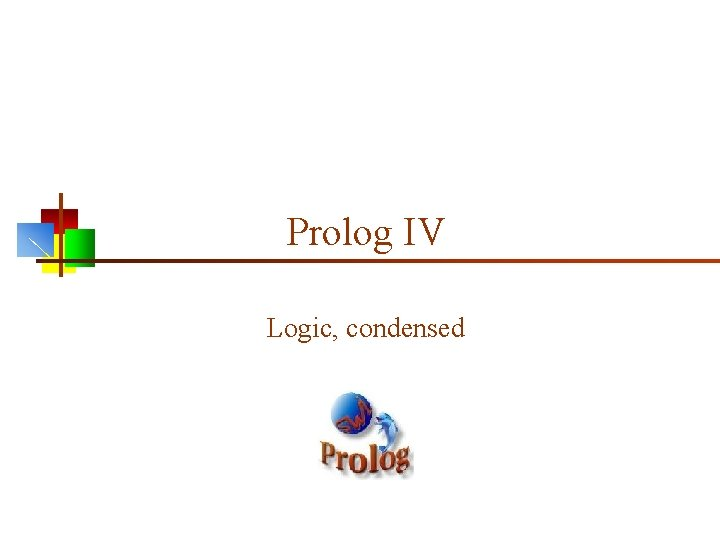Prolog IV Logic, condensedPropositional logic n Propositional logic consists of: n The logical values true and false (T and F) n Propositions: “Sentences, ” which n n n Are atomic (that is, they must be treated as indivisible units, with no internal structure), and Have a single logical value, either true or false Operators, both unary and binary; when applied to logical values, yield logical values n The usual operators are and, or, not, and implies 2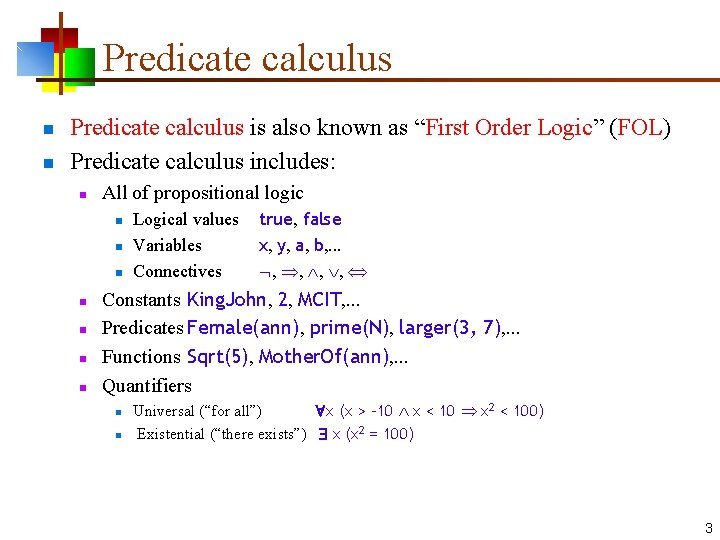Predicate calculus n n Predicate calculus is also known as “First Order Logic” (FOL) Predicate calculus includes: n All of propositional logic n n n n Logical values Variables Connectives true, false x, y, a, b, . . . , , Constants King. John, 2, MCIT, . . . Predicates Female(ann), prime(N), larger(3, 7), . . . Functions Sqrt(5), Mother. Of(ann), . . . Quantifiers n n Universal (“for all”) x (x > -10 x < 10 x 2 < 100) Existential (“there exists”) x (x 2 = 100) 3Clause form n n A clause is a disjunction (“or”) of zero or more literals, some or all of which may be negated Example: sinks(X) dissolves(X, water) ¬denser(X, water) Notice that clauses use only “or” and “not”—they do not use “and, ” “implies, ” or either of the quantifiers “for all” or “there exists” The impressive part is that any predicate calculus expression can be put into clause form 4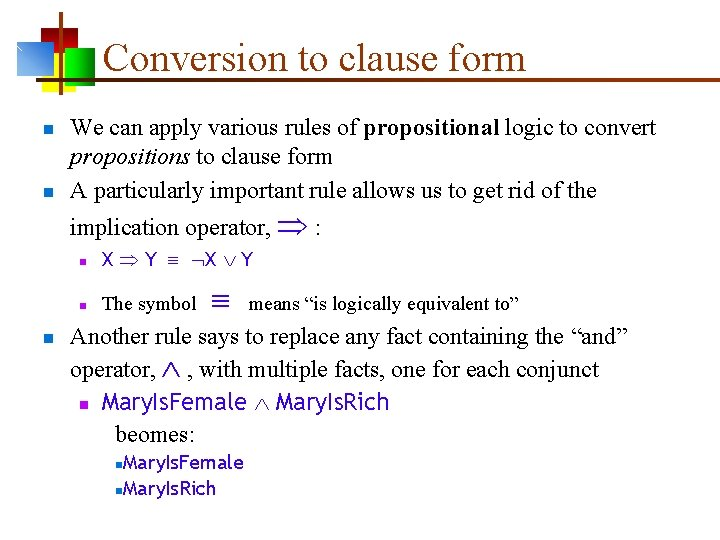Conversion to clause form n n We can apply various rules of propositional logic to convert propositions to clause form A particularly important rule allows us to get rid of the implication operator, : n n X Y n The symbol means “is logically equivalent to” Another rule says to replace any fact containing the “and” operator, , with multiple facts, one for each conjunct n Mary. Is. Female Mary. Is. Rich beomes: Mary. Is. Female n. Mary. Is. Rich nElimination of quantifiers n It’s easy to eliminate “for all”, -- Just drop them, and assume that any variables can stand for (unify with) anything n n We can eliminate “there exists”, , by introducing Skolem functions: n n n x (x > -10 x < 10 x 2 < 100) becomes x > -10 x < 10 x 2 < 100) If x, p(x) then just pick one; call it x’ If the existential quantifier is under control of a universal quantifier, then the picked value has to be a function of the universally quantified variable: n If x, y, p(x, y) then x, p(x, y(x)) These rules can be made into a mechanical process for turning any predicate into clause formThe fact base n A fact base is a collection of “facts, ” expressed in predicate calculus, that are presumed to be true (valid) n These facts are implicitly “anded” together n Example fact base: n seafood(X) likes(John, X) seafood(shrimp) pasta(X) likes(Mary, X) n pasta(spaghetti) n n (where X is a variable) (where X is a different variable; the scope of a variable is a single fact) 7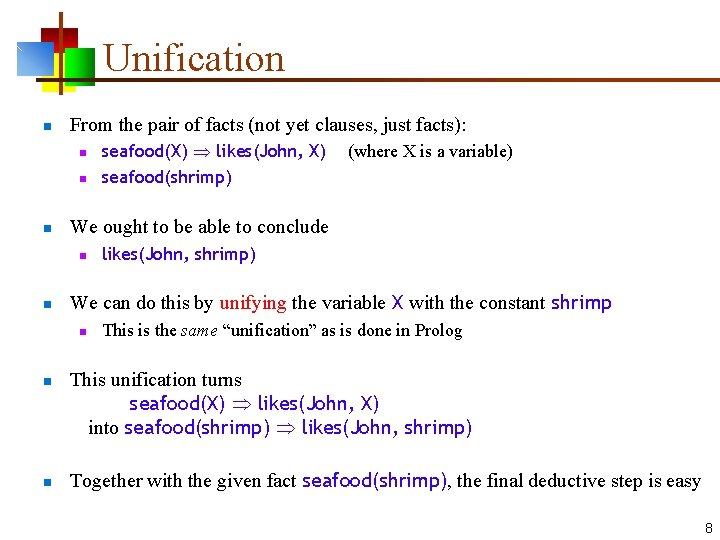Unification n From the pair of facts (not yet clauses, just facts): n n likes(John, shrimp) We can do this by unifying the variable X with the constant shrimp n n (where X is a variable) We ought to be able to conclude n n seafood(X) likes(John, X) seafood(shrimp) This is the same “unification” as is done in Prolog This unification turns seafood(X) likes(John, X) into seafood(shrimp) likes(John, shrimp) Together with the given fact seafood(shrimp), the final deductive step is easy 8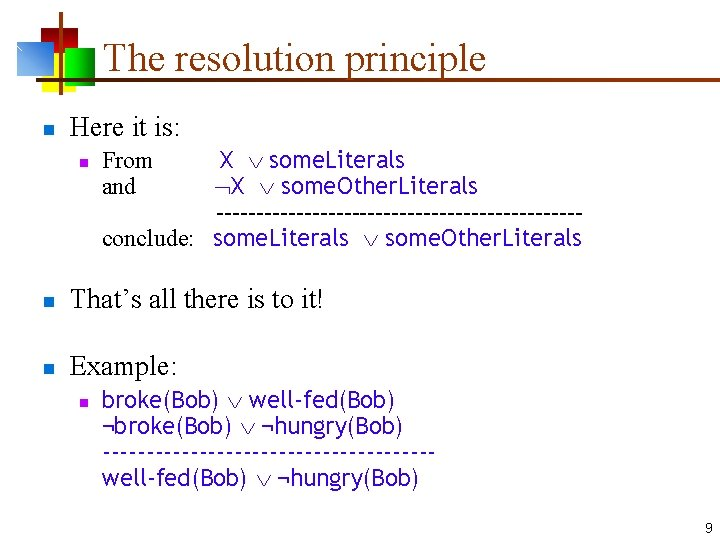The resolution principle n Here it is: n X some. Literals X some. Other. Literals -----------------------conclude: some. Literals some. Other. Literals From and n That’s all there is to it! n Example: n broke(Bob) well-fed(Bob) ¬broke(Bob) ¬hungry(Bob) -------------------well-fed(Bob) ¬hungry(Bob) 9How to prove theorems in logic n Here’s how: n n Transform each fact into clause form Apply the resolution principle to a pair of clauses n n Clauses are closed with respect to resolution--that is, when you resolve two clauses, you get a new clause Add the new clause to your fact base Stop when you have your answer The number of facts grows linearly n n You still have to choose a pair of facts to resolve; but. . . You never have to choose a rule, because there’s only one 10A common error n n You can only do one resolution at a time Example: n n You can resolve on broke to get: n n n well-fed(Bob) happy(Bob) ¬hungry(Bob) ¬happy(Bob) T Or you can resolve on happy to get: n n broke(Bob) well-fed(Bob) happy(Bob) ¬broke(Bob) ¬hungry(Bob) ∨ ¬happy(Bob) broke(Bob) well-fed(Bob) ¬broke(Bob) ¬hungry(Bob) T Note that both legal resolutions yield a tautology (a trivially true statement, containing X ¬X), which is correct but useless But you cannot resolve on both at once to get: n well-fed(Bob) ¬hungry(Bob) 11A first example n “Everywhere that John goes, Rover goes. John is at school. ” n n n We use implication elimination to change the first of these into clause form: n n n at(John, X) at(Rover, X) (not yet in clause form) at(John, school) (already in clause form) at(John, X) at(Rover, X) at(John, school) We can resolve these on at(-, -), but to do so we have to unify X with school; this gives: n at(Rover, school) 12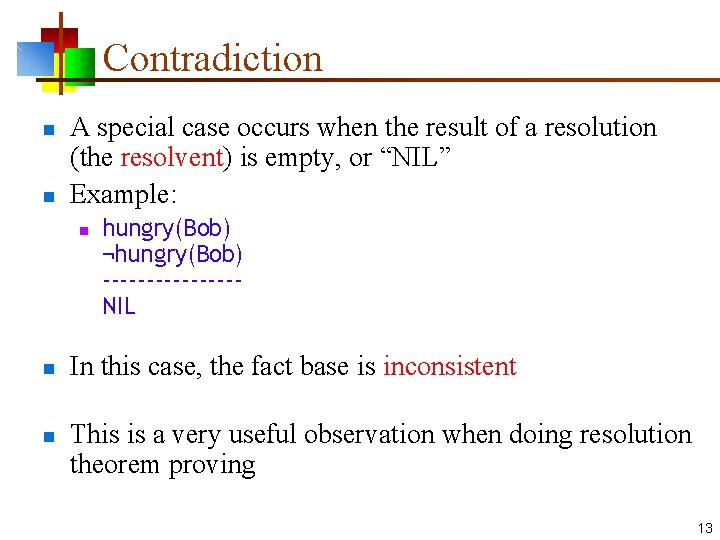Contradiction n n A special case occurs when the result of a resolution (the resolvent) is empty, or “NIL” Example: n n n hungry(Bob) ¬hungry(Bob) --------NIL In this case, the fact base is inconsistent This is a very useful observation when doing resolution theorem proving 13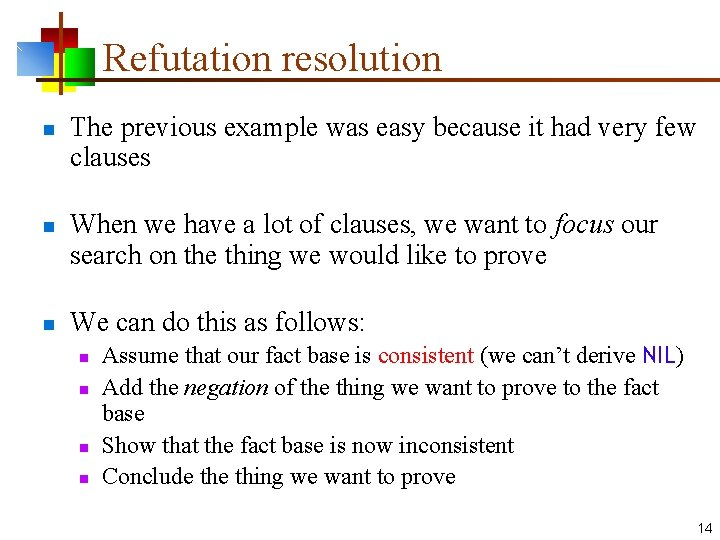Refutation resolution n The previous example was easy because it had very few clauses When we have a lot of clauses, we want to focus our search on the thing we would like to prove We can do this as follows: n n Assume that our fact base is consistent (we can’t derive NIL) Add the negation of the thing we want to prove to the fact base Show that the fact base is now inconsistent Conclude thing we want to prove 14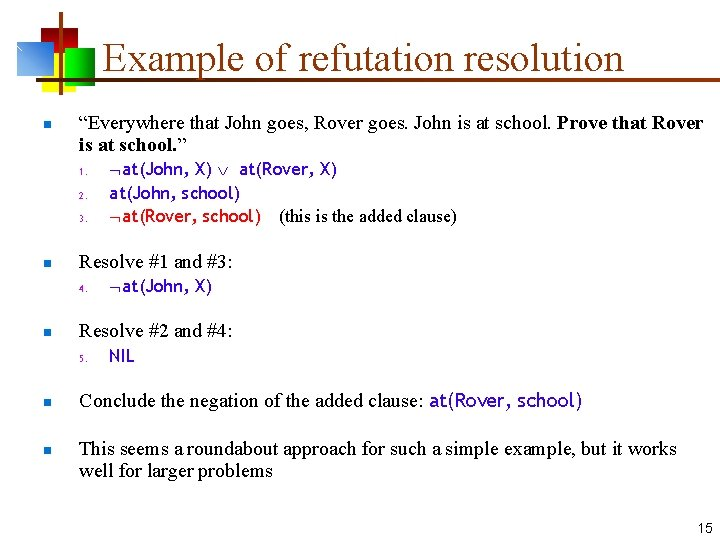Example of refutation resolution n “Everywhere that John goes, Rover goes. John is at school. Prove that Rover is at school. ” 1. 2. 3. n Resolve #1 and #3: 4. n n at(John, X) Resolve #2 and #4: 5. n at(John, X) at(Rover, X) at(John, school) at(Rover, school) (this is the added clause) NIL Conclude the negation of the added clause: at(Rover, school) This seems a roundabout approach for such a simple example, but it works well for larger problems 15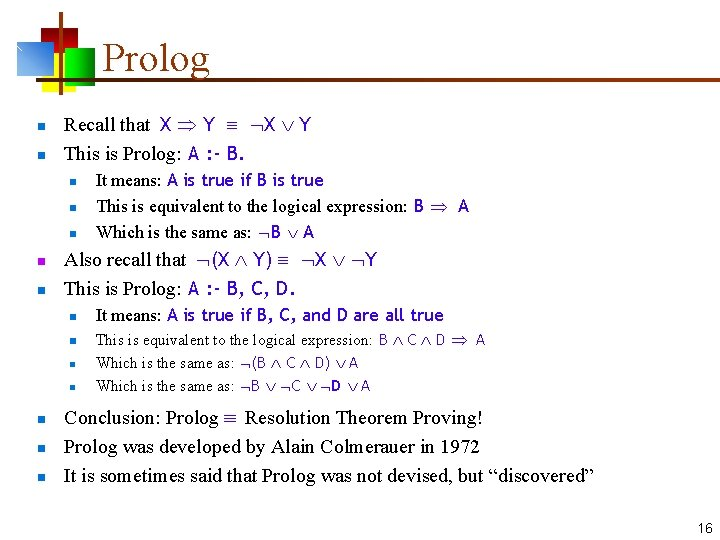Prolog n n Recall that X Y This is Prolog: A : - B. n n n Also recall that (X Y) X Y This is Prolog: A : - B, C, D. n n n n It means: A is true if B is true This is equivalent to the logical expression: B A Which is the same as: B A It means: A is true if B, C, and D are all true This is equivalent to the logical expression: B C D A Which is the same as: (B C D) A Which is the same as: B C D A Conclusion: Prolog Resolution Theorem Proving! Prolog was developed by Alain Colmerauer in 1972 It is sometimes said that Prolog was not devised, but “discovered” 16The End